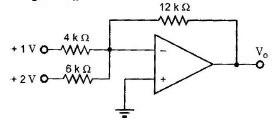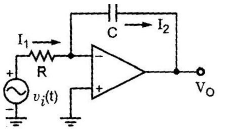Courses

# Test: Basic Electronics - 2

## 25 Questions MCQ Test Electrical Engineering SSC JE (Technical) | Test: Basic Electronics - 2

Description
This mock test of Test: Basic Electronics - 2 for Electrical Engineering (EE) helps you for every Electrical Engineering (EE) entrance exam. This contains 25 Multiple Choice Questions for Electrical Engineering (EE) Test: Basic Electronics - 2 (mcq) to study with solutions a complete question bank. The solved questions answers in this Test: Basic Electronics - 2 quiz give you a good mix of easy questions and tough questions. Electrical Engineering (EE) students definitely take this Test: Basic Electronics - 2 exercise for a better result in the exam. You can find other Test: Basic Electronics - 2 extra questions, long questions & short questions for Electrical Engineering (EE) on EduRev as well by searching above.
QUESTION: 1

Solution:
QUESTION: 2

Solution:
QUESTION: 3

### The shape of the transfer characteristic of JFET is very nearly a

Solution:
QUESTION: 4

In a JFET, drain current is primarily controlled by

Solution:
QUESTION: 5

JFET has main drawback of

Solution:
QUESTION: 6

Consider the following statements:
1. BJT is a current controlled device with a high input impedance and high gain bandwidth
2. FET is a voltage controlled device with high input impedance and low gain bandwidth
3. UJT is a negative resistance device and can be used as an oscillator.
4. BJT, FET and UJT can all be used for amplification.
Which of the statement given above are correct?

Solution:
QUESTION: 7

A Darlington amplifier has a

Solution:
QUESTION: 8

The Darlington pair is mainly used for

Solution:
QUESTION: 9

The BJT amplifier which offers highest input impedance and least voltage gain is

Solution:
QUESTION: 10

The Barkhausen criterion for sustained oscillations is given by

Solution:
QUESTION: 11

Oscillators use following feedback.

Solution:
QUESTION: 12

Most important advantage of an IC is its

Solution:
QUESTION: 13

Ultraviolet radiation is used in IC fabrication process for

Solution:
QUESTION: 14

Why is silicon dioxide (SiO2) layer used in ICs ?

Solution:
QUESTION: 15

An ideal op-amp is an ideal

Solution:
QUESTION: 16

The ideal op-amp has the following characteristics.

Solution:
QUESTION: 17

An op-amp has a common mode gain of 0.01 and a differential mode gain of 105. Its CMRR would be

Solution:
QUESTION: 18

Which one of the following causes phase shift through causes phase shift through an op-amp?

Solution:
QUESTION: 19

In the circuit shown in the figure, the value of output 'V0' isSolution:
QUESTION: 20

For the circuit shown in figure. given by the output voltage V0 isSolution:
QUESTION: 21

A sinusoidal waveform can be converted to a square waveform by

Solution:
QUESTION: 22

Noise on the input of the comparator can cause the output to

Solution:
QUESTION: 23

The zero level detector is one application of a

Solution:
QUESTION: 24

How are the zener diodes placed internally in MOSFETs so as to provide defence from the hazardous consequences of stray voltages?

Solution:
QUESTION: 25

A device having characteristies very close to that of an ideal voltage source is

Solution: